# 前言

## 編輯小語

---- （「少年科技人雜誌」與「程式人雜誌」編輯 - 陳鍾誠）

## 授權聲明

1. 標示原作者姓名 (包含該文章作者，若有來自維基百科的部份也請一併標示)。
2. 採用 創作共用：姓名標示、相同方式分享 的方式公開衍生著作。

# 本期焦點： Nand2Tetris 慕課記 -- 從邏輯閘到方塊遊戲

## 慕課記 -- Nand2Tetris 這門課

### 慕課 MOOC 是什麼？

Massive open online course 簡稱 MOOC ，這是一個從 2008 年 Dave Cormier 提出來的新名詞，其內涵是運用網路技術讓大家可以共同學習一門課程，我們曾經在下列的程式人雜誌文章當中介紹過這個主題。

MOOC 這個名詞在台灣通常翻譯成『磨課師』，而在中國則翻譯成『慕課』，我本身比較喜歡『慕課』這個譯法。因為『慕課』感覺就像『很羨慕的來上課』的樣子，而『磨課師』則要由老師磨來磨去，聽起來就不太開心。

Coursera 是由史丹佛大學的計算機科學教授吳恩達 （Andrew Ng） 和 Daphne Koller 聯合創建的，目前台灣大學也有一些課程在 Coursera 開設，像是葉丙成老師的機率，還有呂世浩老師的秦始皇等等，都是相當受歡迎的課程。

### 令人心動的 From Nand to Tetris 課程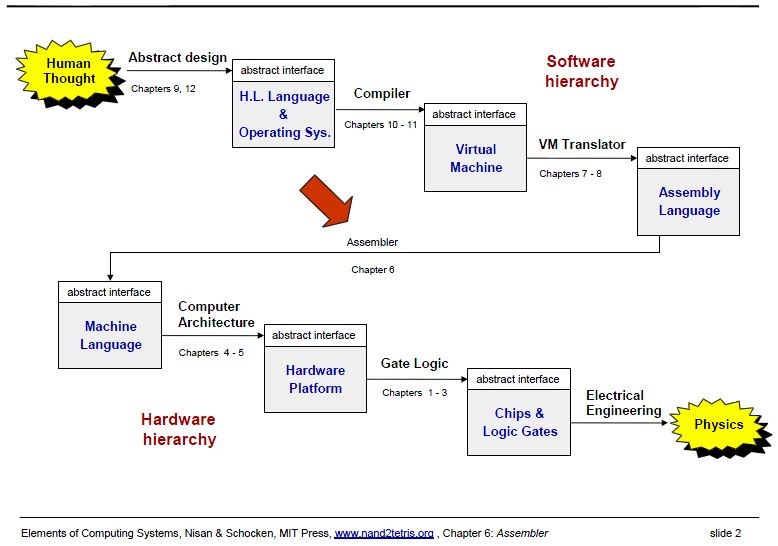### 開始修課吧！

``````0. From Nand to Tetris
1. Boolean Functions
2. Boolean Arithmetic
3. Memory
4. Machine Language
5. Computer Architecture
6. Assembler``````

Part II 應該會有下列主題。

``````7. VM I: Stack Arithmetic
8. VM II: Program Control
9. High-Level Language Project
10. Compiler I: Syntax Analysis Project
11. Compiler II: Code Generation Project
12. Operating System``````

## Nand2Tetris 第 0 週 -- 課程簡介與的軟體安裝

### 課程簡介

From Nand to Tetris / Part I 這門課總共有七週，分為第 0 週到第 6 週，第 0 週的內容是課程簡介和軟體安裝，真正的實作還是從第 1 週開始的。

### 軟體安裝

``````nqu-192-168-61-142:nand2tetris mac020\$ cd tools
nqu-192-168-61-142:tools mac020\$ ls
Assembler.bat       JackCompiler.bat    VMEmulator.sh
Assembler.sh        JackCompiler.sh     bin
CPUEmulator.bat     OS          builtInChips
CPUEmulator.sh      TextComparer.bat    builtInVMCode
HardwareSimulator.bat   TextComparer.sh
HardwareSimulator.sh    VMEmulator.bat``````

HardwareSimulator 是用來測試我們所寫的『 HDL 硬體描述語言』所使用的，此處的『硬體描述語言』 並不是常見的 Verilog 或 VHDL，而是 nand2tetris 團隊所設計出來的『簡易陽春版 HDL』，稱為 HackHDL 。

``````// This file is part of www.nand2tetris.org
// and the book "The Elements of Computing Systems"
// by Nisan and Schocken, MIT Press.
// File name: projects/01/And16.hdl

/**
* 16-bit bitwise And:
* for i = 0..15: out[i] = (a[i] and b[i])
*/

CHIP And16 {
IN a, b;
OUT out;

PARTS:
}``````

``for i = 0..15: out[i] = (a[i] and b[i])``

``````// This file is part of www.nand2tetris.org
// and the book "The Elements of Computing Systems"
// by Nisan and Schocken, MIT Press.

/**
*  Exclusive-or gate: out = !(a == b).
*/

CHIP Xor {

IN a, b;
OUT out;

PARTS:
Nand (a=a, b=b, out= AnandB);
Or   (a=a, b=b, out= AorB);
And  (a=AnandB, b=AorB, out=out);
}``````

``````Code Posting Policy

Therefore, we request that you don't post solutions publicly on the web, e.g. in blogs or forums. If your course instructor or organizer creates a private space in which work can be shared outside the public domain, that's fine. Likewise, you can share your work with others using a password-protected space, if it's permitted by the specific course in which you are enrolled.``````

## Nand2Tetris 第 1 週 -- 布林函數

### 電腦的基本結構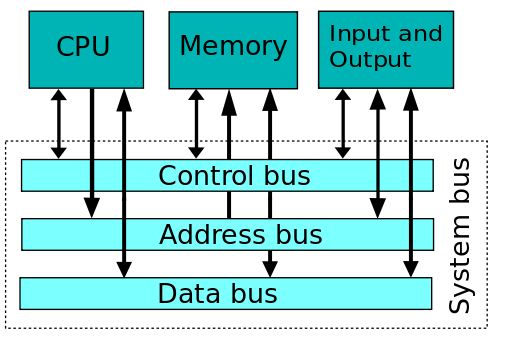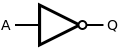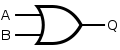### 如何設計電腦

Shimon Schocken 與 Noam Nisan 兩位教授應該是希望透過這樣一個從下到上的完整建構過程，讓學生能夠徹底的瞭解電腦的設計原理，並且能自己動手設計出一台電腦。

1. 問題是，組合出一顆處理器需要多少閘呢？

1. 這樣的話，我們花一輩子都可能組不出來啊？那我們要怎麼開始呢？

1. 寫程式來設計電腦，那要用什麼程式語言呢？

1. 那在這一門課裡，我們是用哪種硬體描述語言呢？是 VHDL 還是 Verilog 呢？

1. HackHDL 和 VHDL, Verilog 有差別嗎？差別在哪裡呢？

1. 為什麼要採用這麼陽春的工具，這對我們的學習是不是會有負面影響？

### 硬體描述語言

``````CHIP Not {
IN in;
OUT out;

PARTS:
Nand(a=in, b=in, out=out);
}``````

``````CHIP Nand {
IN a, b;
OUT out;

BUILTIN Nand; // 使用內建的 Nand 功能
}``````

``````CHIP Nand {
IN a, b;
OUT out;

PARTS:
Nand(a=a, b=b, out=out);
}``````

### 執行方法### 使用 nand 兜出基本邏輯閘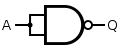``````CHIP Not {
IN in;
OUT out;

PARTS:
}``````

``    Nand(a=in, b=in, out=out);````````CHIP And {
IN a, b;
OUT out;

PARTS:
}````````````CHIP Or {
IN a, b;
OUT out;

PARTS:
Not(in=a, out=nota);
Not(in=b, out=notb);
Nand(a=nota, b=notb, out=out);
}``````

``````CHIP Xor {
IN a, b;
OUT out;

PARTS:
}````````````CHIP Mux {
IN a, b, sel;
OUT out;

PARTS:
}``````

``````CHIP DMux {
IN in, sel;
OUT a, b;

PARTS:
}``````

``````/**
* 16-bit Not:
* for i=0..15: out[i] = not in[i]
*/

CHIP Not16 {
IN in;
OUT out;

PARTS:
}``````
``````/**
* 16-bit bitwise And:
* for i = 0..15: out[i] = (a[i] and b[i])
*/

CHIP And16 {
IN a, b;
OUT out;

PARTS:
}``````
``````/**
* 16-bit bitwise Or:
* for i = 0..15 out[i] = (a[i] or b[i])
*/

CHIP Or16 {
IN a, b;
OUT out;

PARTS:
}``````
``````/**
* 16-bit multiplexor:
* for i = 0..15 out[i] = a[i] if sel == 0
*                        b[i] if sel == 1
*/

CHIP Mux16 {
IN a, b, sel;
OUT out;

PARTS:
}``````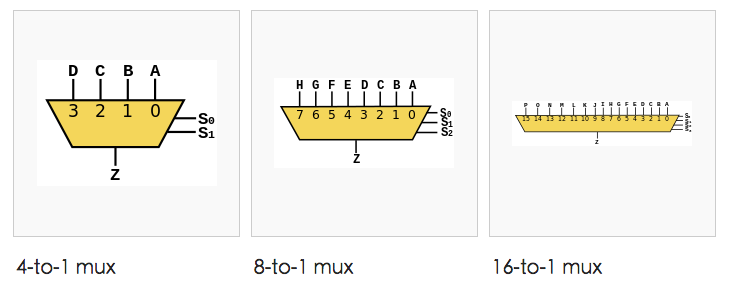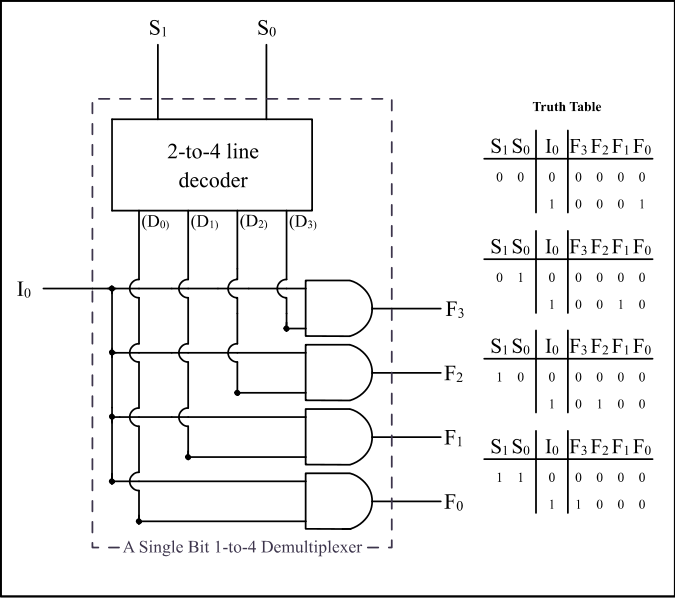``````/**
* 4-way 16-bit multiplexor:
* out = a if sel == 00
*       b if sel == 01
*       c if sel == 10
*       d if sel == 11
*/

CHIP Mux4Way16 {
IN a, b, c, d, sel;
OUT out;

PARTS:
}``````
``````/**
* 8-way 16-bit multiplexor:
* out = a if sel == 000
*       b if sel == 001
*       etc.
*       h if sel == 111
*/

CHIP Mux8Way16 {
IN a, b, c, d,
e, f, g, h,
sel;
OUT out;

PARTS:
}``````
``````/**
* 4-way demultiplexor:
* {a, b, c, d} = {in, 0, 0, 0} if sel == 00
*                {0, in, 0, 0} if sel == 01
*                {0, 0, in, 0} if sel == 10
*                {0, 0, 0, in} if sel == 11
*/

CHIP DMux4Way {
IN in, sel;
OUT a, b, c, d;

PARTS:
}``````
``````/**
* 8-way demultiplexor:
* {a, b, c, d, e, f, g, h} = {in, 0, 0, 0, 0, 0, 0, 0} if sel == 000
*                            {0, in, 0, 0, 0, 0, 0, 0} if sel == 001
*                            etc.
*                            {0, 0, 0, 0, 0, 0, 0, in} if sel == 111
*/

CHIP DMux8Way {
IN in, sel;
OUT a, b, c, d, e, f, g, h;

PARTS:
}``````

``````/**
* 8-way Or:
* out = (in or in or ... or in)
*/

CHIP Or8Way {
IN in;
OUT out;

PARTS:
}``````

## Nand2Tetris 第 2 週 -- 布林算術``````/**
* Computes the sum of two bits.
*/

IN a, b;    // 1-bit inputs
OUT sum,    // Right bit of a + b
carry;  // Left bit of a + b

PARTS:
// Put you code here:
}````````````/**
* Computes the sum of three bits.
*/

IN a, b, c;  // 1-bit inputs
OUT sum,     // Right bit of a + b + c
carry;   // Left bit of a + b + c

PARTS:
// Put you code here:
}``````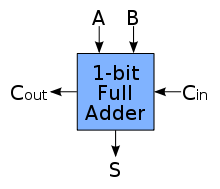``````/**
* The most significant carry bit is ignored.
*/

IN a, b;
OUT out;

PARTS:
// Put you code here:
}``````

``````/**
* 16-bit incrementer:
* out = in + 1 (arithmetic addition)
*/

CHIP Inc16 {
IN in;
OUT out;

PARTS:
// Put you code here:
}``````

``````/**
* The ALU (Arithmetic Logic Unit).
* Computes one of the following functions:
* x+y, x-y, y-x, 0, 1, -1, x, y, -x, -y, !x, !y,
* x+1, y+1, x-1, y-1, x&y, x|y on two 16-bit inputs,
* according to 6 input bits denoted zx,nx,zy,ny,f,no.
* In addition, the ALU computes two 1-bit outputs:
* if the ALU output == 0, zr is set to 1; otherwise zr is set to 0;
* if the ALU output < 0, ng is set to 1; otherwise ng is set to 0.
*/

// Implementation: the ALU logic manipulates the x and y inputs
// and operates on the resulting values, as follows:
// if (zx == 1) set x = 0        // 16-bit constant
// if (nx == 1) set x = !x       // bitwise not
// if (zy == 1) set y = 0        // 16-bit constant
// if (ny == 1) set y = !y       // bitwise not
// if (f == 1)  set out = x + y  // integer 2's complement addition
// if (f == 0)  set out = x & y  // bitwise and
// if (no == 1) set out = !out   // bitwise not
// if (out == 0) set zr = 1
// if (out < 0) set ng = 1

CHIP ALU {
IN
x, y,  // 16-bit inputs
zx, // zero the x input?
nx, // negate the x input?
zy, // zero the y input?
ny, // negate the y input?
f,  // compute out = x + y (if 1) or x & y (if 0)
no; // negate the out output?

OUT
out, // 16-bit output
zr, // 1 if (out == 0), 0 otherwise
ng; // 1 if (out < 0),  0 otherwise

PARTS:
// Put you code here:
}``````

### 注意事項

``````Sub-busing can only be used on buses that are named in the IN and OUT statements of an HDL file, or inputs and outputs of the chip-parts used in the PARTS section. If you need a sub-bus of an internal bus, you must create the narrower bus as an output from a chip-part. For example:

CHIP Foo {
IN in;
OUT out;
PARTS:
Something16 (in=in, out=notIn);
Or8Way (in=notIn[4..11], out=out);
}

This implementation causes an error on the Or8Way statement. This needs to be coded as:

Something16 (in=in, out[4..11]=notIn);
Or8Way (in=notIn, out=out);

Multiple Outputs

Sometimes you need more than one sub-bus connected to the output of a chip-part. Simply add more than one out= connection to the chip-part definition.

CHIP Foo {
IN in;
OUT out;
PARTS:
Not16 (in=in, out[0..7]=low8, out[8..15]=high8);
Something8 (a=low8, b=high8, out=out);
}

This also works if you want to use an output of a chip in further computations.

CHIP Foo {
IN a, b, c;
OUT out1, out2;
PARTS:
Something (a=a, b=b, out=x, out=out1);
Whatever (a=x, b=c, out=out2);
}``````

## Nand2Tetris 第 3 週 -- 記憶體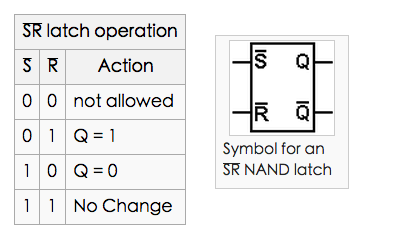Nand2tetris 課程內建了 D 型的正反器元件，稱為 DFF，您可以透過 DFF 來組成一位元記憶體，而不需要從 NAND 開始建構。

``````/**
* 1-bit register:
* If load[t] == 1 then out[t+1] = in[t]
*                 else out does not change (out[t+1] = out[t])
*/

CHIP Bit {
OUT out;

PARTS:
}
``````

``````/**
* 16-bit register:
* If load[t] == 1 then out[t+1] = in[t]
* else out does not change
*/

CHIP Register {
OUT out;

PARTS:
}``````

``````/**
* Memory of 8 registers, each 16 bit-wide. Out holds the value
* stored at the memory location specified by address. If load==1, then
* the in value is loaded into the memory location specified by address
* (the loaded value will be emitted to out from the next time step onward).
*/

CHIP RAM8 {
OUT out;

PARTS:
}``````
``````/**
* Memory of 64 registers, each 16 bit-wide. Out holds the value
* stored at the memory location specified by address. If load==1, then
* the in value is loaded into the memory location specified by address
* (the loaded value will be emitted to out from the next time step onward).
*/

CHIP RAM64 {
OUT out;

PARTS:
}``````
``````/**
* Memory of 512 registers, each 16 bit-wide. Out holds the value
* stored at the memory location specified by address. If load==1, then
* the in value is loaded into the memory location specified by address
* (the loaded value will be emitted to out from the next time step onward).
*/

CHIP RAM512 {
OUT out;

PARTS:
}``````
``````/**
* Memory of 4K registers, each 16 bit-wide. Out holds the value
* stored at the memory location specified by address. If load==1, then
* the in value is loaded into the memory location specified by address
* (the loaded value will be emitted to out from the next time step onward).
*/

CHIP RAM4K {
OUT out;

PARTS:
}``````
``````/**
* Memory of 16K registers, each 16 bit-wide. Out holds the value
* stored at the memory location specified by address. If load==1, then
* the in value is loaded into the memory location specified by address
* (the loaded value will be emitted to out from the next time step onward).
*/

CHIP RAM16K {
OUT out;

PARTS:
}``````

## Nand2Tetris 第 4 週 -- 機器語言

### HackCPU 的組合語言

``````    // Adds 1+...+10.
@i // i refers to some RAM location
M=1 // i=1
@sum // sum refers to some RAM location
M=0 // sum=0
(LOOP)
@i
D=M // D = i
@10
D=D-A // D = i - 10
@END
D;JGT // If (i-100) > 0 goto END
@i
D=M // D = i
@sum
M=D+M // sum += i
@i
M=M+1 // i++
@LOOP
0;JMP // Got LOOP
(END)
@END
0;JMP // Infinite loop``````

### 指令格式與寫法

Nand2tetris 課程所要求要設計的處理器稱為 HackCPU，HackCPU 的有兩種型態的指令，第一種是 A 型態的指令，用來指定某個記憶體位址， A 型指令的寫法是由 @ 符號開頭的，如下所示：

``@value // 代表 A=value``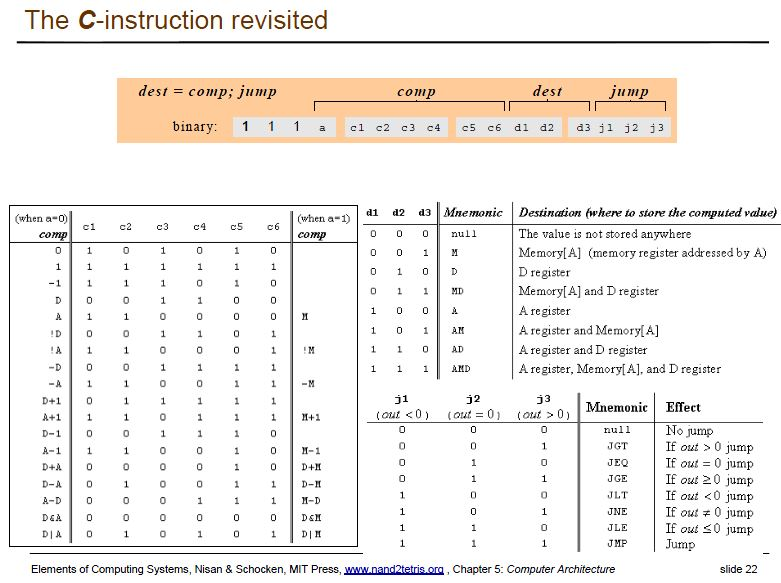### 範例

``````@3      // A = 3
D = M   // D = M = memory[A] = memory
@5      // A = 5
D = D-A // D = D-5
@100    // A = 100
D; JEQ  // if (D==0) goto 100      ; goto 會跳到 A 暫存器的位址。
@200    // A = 200
0; JMP  // goto 200``````

### 符號與記憶體映射### 第四週習題

``````// Runs an infinite loop that listens to the keyboard input.
// When a key is pressed (any key), the program blackens the screen,
// i.e. writes "black" in every pixel. When no key is pressed, the
// program clears the screen, i.e. writes "white" in every pixel.

``````// Multiplies R0 and R1 and stores the result in R2.
// (R0, R1, R2 refer to RAM, RAM, and RAM, respectively.)

## Nand2Tetris 第 5 週 -- 計算機結構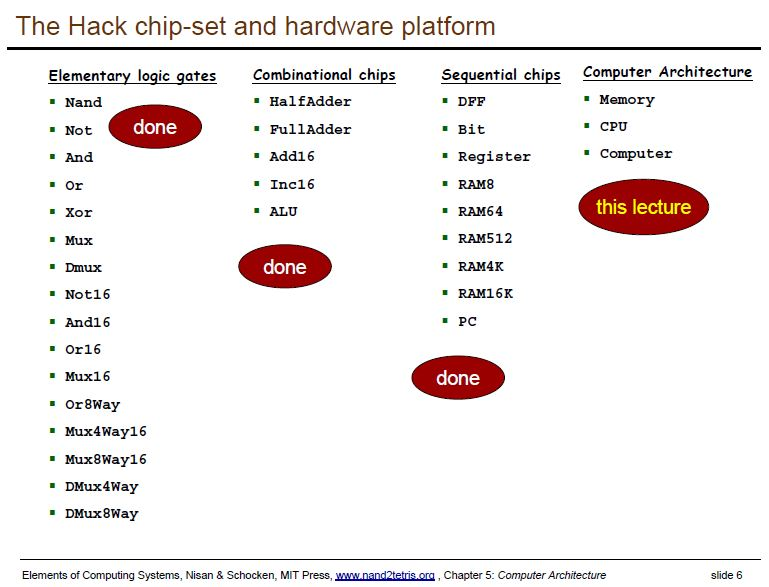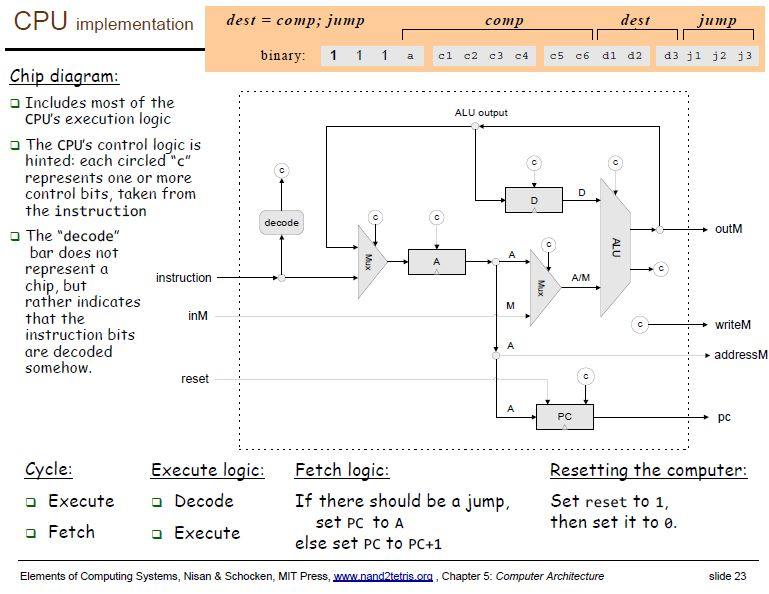``````CHIP CPU {

IN  inM,         // M value input  (M = contents of RAM[A])
instruction, // Instruction for execution
reset;           // Signals whether to re-start the current
// program (reset==1) or continue executing
// the current program (reset==0).

OUT outM,        // M value output
writeM,          // Write to M?
pc;          // address of next instruction

PARTS:
}``````

HackComputer 包含『指令記憶體、資料記憶體和輸出入記憶體』等三部份，以下圖片顯示了 HackCPU 與記憶體的連接方式。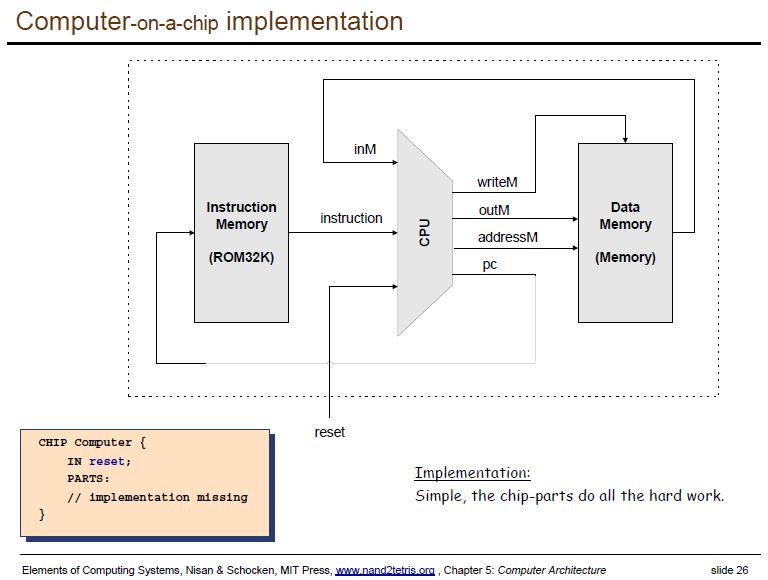``````CHIP Memory {
OUT out;

PARTS:
}``````

``````CHIP Computer {

IN reset;

PARTS:
}``````

## Nand2Tetris 第 6 週 -- 組譯器

``````0000000000010000 1110111111001000 0000000000010001 1110101010001000
0000000000010000 1111110000010000 0000000000001010 1110010011010000
0000000000010010 1110001100000001 0000000000010000 1111110000010000
0000000000010001 1111000010001000 0000000000010000 1111110111001000
0000000000000100 1110101010000111 0000000000010010 1110101010000111``````

``````    // Adds 1+...+10.
@i // i refers to some RAM location
M=1 // i=1
@sum // sum refers to some RAM location
M=0 // sum=0
(LOOP)
@i
D=M // D = i
@10
D=D-A // D = i - 10
@END
D;JGT // If (i-100) > 0 goto END
@i
D=M // D = i
@sum
M=D+M // sum += i
@i
M=M+1 // i++
@LOOP
0;JMP // Got LOOP
(END)
@END
0;JMP // Infinite loop``````

``````nqu-192-168-61-142:n2t mac020\$ iojs ash sum
============== pass1 ================
002:0000    @i // i refers to some RAM location
003:0001    M=1 // i=1
004:0002    @sum // sum refers to some RAM location
005:0003    M=0 // sum=0
symbol: LOOP 0004
007:0004    @i
008:0005    D=M // D = i
009:0006    @10
010:0007    D=D-A // D = i - 10
011:0008    @END
012:0009    D;JGT // If (i-100) > 0 goto END
013:0010    @i
014:0011    D=M // D = i
015:0012    @sum
016:0013    M=D+M // sum += i
017:0014    @i
018:0015    M=M+1 // i++
019:0016    @LOOP
020:0017    0;JMP // Got LOOP
symbol: END 0018
022:0018    @END
023:0019    0;JMP // Infinite loop
============== pass2 ================
002:0000000000010000    @i // i refers to some RAM location
003:1110111111001000    M=1 // i=1
004:0000000000010001    @sum // sum refers to some RAM location
005:1110101010001000    M=0 // sum=0
007:0000000000010000    @i
008:1111110000010000    D=M // D = i
009:0000000000001010    @10
010:1110010011010000    D=D-A // D = i - 10
011:0000000000010010    @END
012:1110001100000001    D;JGT // If (i-100) > 0 goto END
013:0000000000010000    @i
014:1111110000010000    D=M // D = i
015:0000000000010001    @sum
016:1111000010001000    M=D+M // sum += i
017:0000000000010000    @i
018:1111110111001000    M=M+1 // i++
019:0000000000000100    @LOOP
020:1110101010000111    0;JMP // Got LOOP
022:0000000000010010    @END
023:1110101010000111    0;JMP // Infinite loop``````

``````function parse(line, i) {
}

}

function pass1(lines) {
}``````

``````function pass2(lines, objFile) {
}

function toCode(p) {
}``````

``````function assemble(asmFile, objFile) {
var asmText = fs.readFileSync(asmFile, "utf8"); // 讀取檔案到 text 字串中
var lines   = asmText.split(/\r?\n/); // 將組合語言分割成一行一行
pass1(lines);
pass2(lines, objFile);
} ``````

# 雜誌訊息

## 公益資訊

02-27190408分機 232

04-23058005